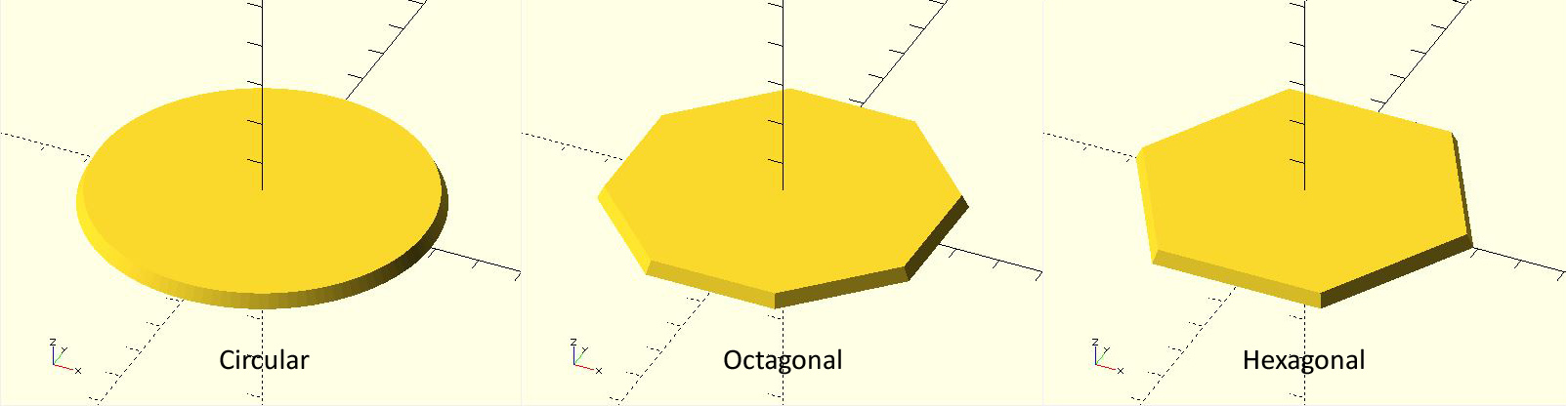The goal of our first program is to create the dial base.  We'll define the dial base parametrically using three parameters for size: dial_btm, dial_top and dial_hght.  This allows for the diameter of the base bottom and top to create tapered sides and base  height.

Normally we think of making a cylinder using an OpenSCAD function called cylinder(h, d) where h is the height and d is the diameter. There is an extension of parameters such that the cylinder can be tapered using cylinder(h,d1,d2) where d1 is the base diameter and d2 is the top diameter.

But suppose that we want to make the sundial base in the shape of a hexagon or octagon.  We rely on a special OpenSCAD variable $fa that sets the minimum angle of our solid. Two or three degrees on the edge makes a nice circle, 45 degrees for each side makes an octagon and 60 degrees for each side makes a hexagon. It makes sense that we could invoke the cylinder functionfor a tapered hexagon as: cylinder($fa =45,h,d1,d2);

We'd like to invoke "if" statements to decide which shape to make.  The code takes the form of

if(shape=="hexagon") { make our tapered cylinder look like a hexagon }The "if" statements (as well as "for") statements in OpenSCAD are handled a bit different than normal code.  Whatever happens within the "if" or "for" statement stays within it.  Variables can't leak out. Therefore we must build the sundial base within each selection of the choices "circular", "hexagon", or "octagon".   Thinking of terms of modular procedures, we can invoke a module called "sundial_base" that takes the appropriate parameters to create one of these three base shapes.

In the attached exemplar code, the size variables of the base (dial_btm, dial_top, dial_hght) from the main program are used directly within the "if" statement as well as within the sundial_base module. The hierarchy of variable scope allows these mainl variables to "fall" into these subservient functions and procedures.  However, within each "if" statement we need to set local paramaters to properly create the desired shape.  So within each "if" statement we create two variables to (1) set minimum segment angle and (2) rotate the base such that the hexagon or octagon has a flat side parallel to the x-axis.

The necessary rotation of the sundial base shows another peculiarity of the OpenSCAD code.  In mathematics one writes the translation or rotation operator (a matrix) to the left of the object being changed.  OpenSCAD does this by writing the operator on the lines before the object.  And there is a subtle use of the ";" symbol to indicate the end of operation:

rotate([xturn,yturn,zturn])          //Note that there is no ";"
cylinder(\$fa,h,d1,d2);               //Specifically ending with ";"